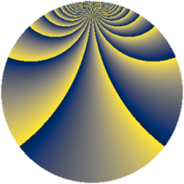# Properties

 Label 1840.2.daLevel $1840$ Weight $2$ Character orbit 1840.da Rep. character $\chi_{1840}(37,\cdot)$ Character field $\Q(\zeta_{44})$ Dimension $5680$ Sturm bound $576$

# Related objects

## Defining parameters

 Level: $$N$$ $$=$$ $$1840 = 2^{4} \cdot 5 \cdot 23$$ Weight: $$k$$ $$=$$ $$2$$ Character orbit: $$[\chi]$$ $$=$$ 1840.da (of order $$44$$ and degree $$20$$) Character conductor: $$\operatorname{cond}(\chi)$$ $$=$$ $$1840$$ Character field: $$\Q(\zeta_{44})$$ Sturm bound: $$576$$

## Dimensions

The following table gives the dimensions of various subspaces of $$M_{2}(1840, [\chi])$$.

Total New Old
Modular forms 5840 5840 0
Cusp forms 5680 5680 0
Eisenstein series 160 160 0

## Trace form

 $$5680q - 18q^{2} + 8q^{4} - 22q^{5} - 36q^{6} - 18q^{8} + 552q^{9} + O(q^{10})$$ $$5680q - 18q^{2} + 8q^{4} - 22q^{5} - 36q^{6} - 18q^{8} + 552q^{9} - 22q^{10} - 44q^{11} - 30q^{12} - 44q^{15} - 28q^{16} - 44q^{17} - 22q^{18} - 22q^{20} - 44q^{21} - 24q^{24} - 36q^{26} - 22q^{28} - 22q^{30} - 72q^{31} + 2q^{32} - 44q^{33} + 2q^{35} - 36q^{36} - 22q^{38} - 48q^{39} - 22q^{40} - 242q^{42} - 44q^{43} + 264q^{44} - 24q^{46} - 80q^{47} + 54q^{48} + 2q^{50} - 44q^{51} - 250q^{52} - 44q^{53} - 40q^{54} - 44q^{56} - 42q^{58} - 22q^{60} - 44q^{61} - 66q^{62} - 44q^{63} + 32q^{64} - 44q^{65} - 44q^{66} - 44q^{67} + 4q^{69} - 28q^{70} + 68q^{72} - 30q^{75} - 44q^{76} + 20q^{77} + 6q^{78} - 22q^{80} - 576q^{81} - 26q^{82} + 2q^{85} - 44q^{86} - 24q^{87} - 22q^{88} + 22q^{90} + 54q^{92} + 8q^{94} - 36q^{95} - 36q^{96} - 44q^{97} - 82q^{98} + O(q^{100})$$

## Decomposition of $$S_{2}^{\mathrm{new}}(1840, [\chi])$$ into newform subspaces

The newforms in this space have not yet been added to the LMFDB.